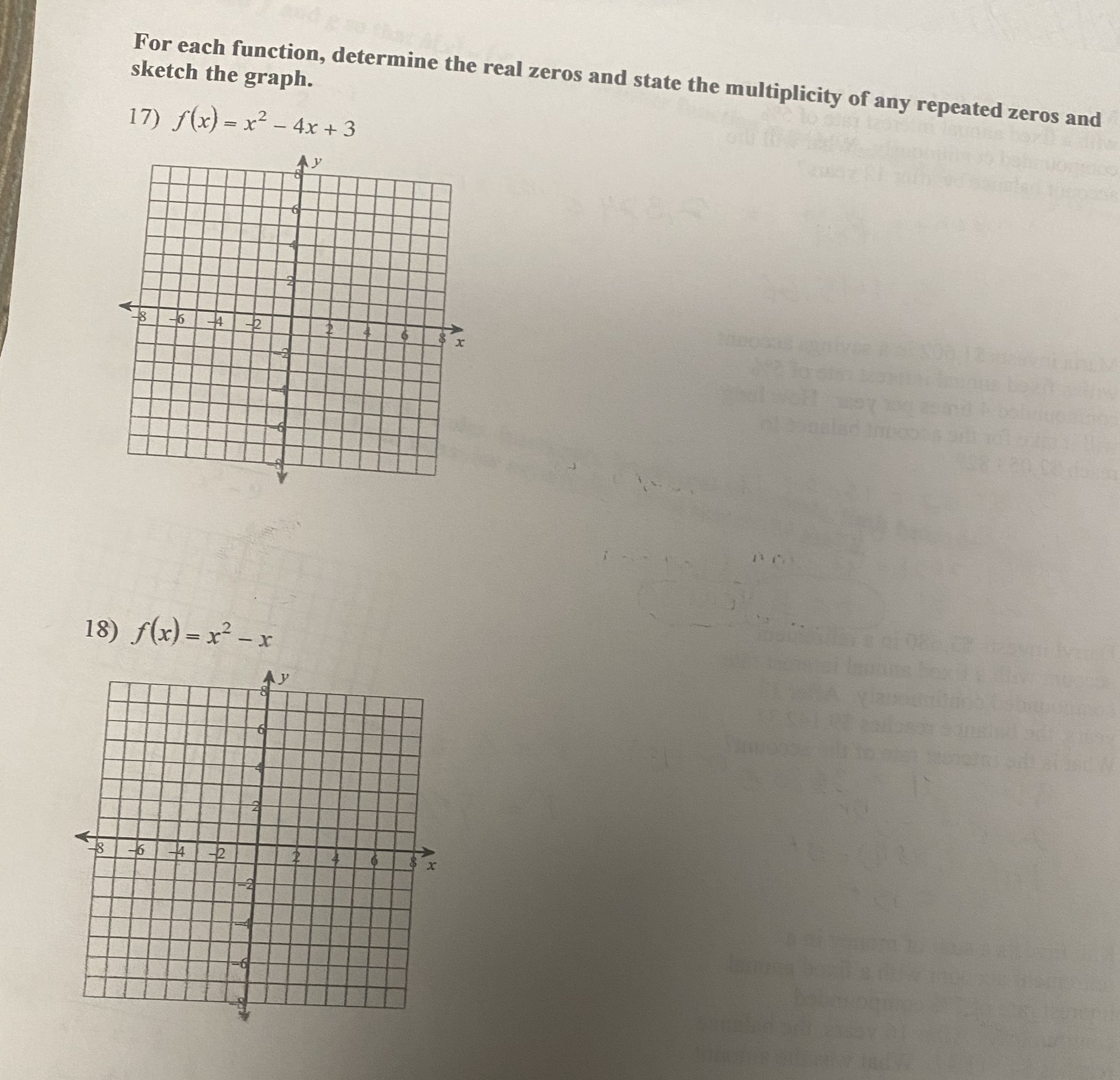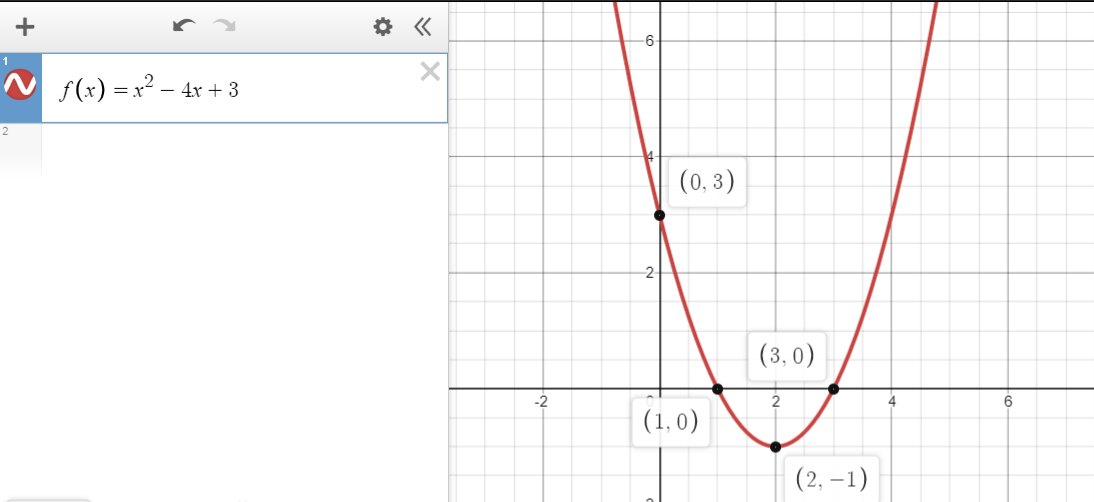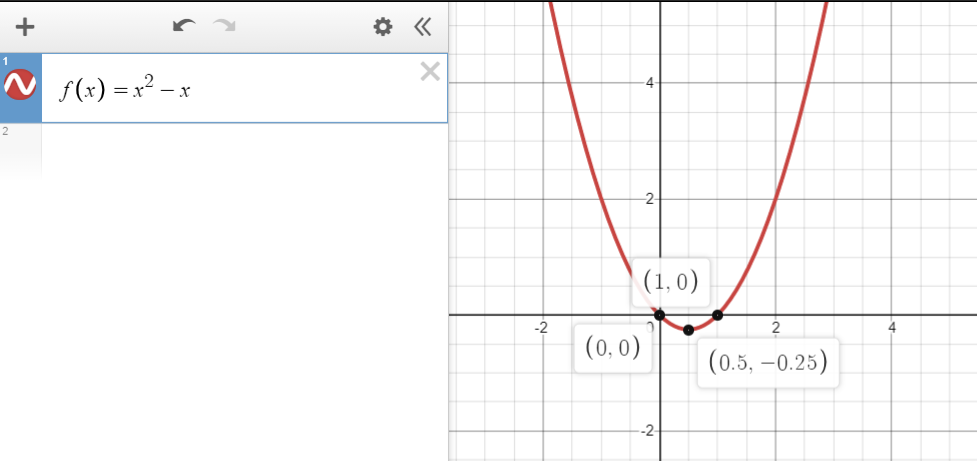### Still have math questions?

Algebra
QuestionFor each function, determine the real zeros and state the multiplicity of any repeated zeros and sketch the graph.

17) $$f ( x ) = x ^ { 2 } - 4 x + 3$$

18) $$f ( x ) = x ^ { 2 } - x$$